# Impulse predictors for epochs

`epoch_impulse_predictor()` generates predictor variables for reverse correlation in trial-based experiments with discrete events. The function generates one impulse per trial, and these impulsescan be of varaiable magnitude and have variable latency.

The example uses simulated data meant to vaguely resemble data from an N400 experiment, but not intended as a physiologically realistic simulation.

```# sphinx_gallery_thumbnail_number = 2
from eelbrain import *

ds = datasets.simulate_erp(snr=1)
print(ds.summary())
```
```Key              Type     Values
-------------------------------------------------------------------------
eeg              NDVar    140 time, 65 sensor; -8.21333e-06 - 8.36563e-06
cloze            Var      0.00563694 - 0.997675
predictability   Factor   high:40, low:40
n_chars          Var      3:10, 4:20, 5:22, 6:16, 7:12
-------------------------------------------------------------------------
Dataset: 80 cases
```

## Discrete events

Computing a TRF for an impulse at trial onset is very similar to averaging:

```any_trial = epoch_impulse_predictor('eeg', 1, data=ds)
fit = boosting('eeg', any_trial, -0.100, 0.600, basis=0.050, data=ds, partitions=2, delta=0.01)
average = ds['eeg'].mean('case')
trf = fit.h.sub(time=(average.time.tmin, average.time.tstop))
p = plot.TopoButterfly([fit.h_scaled, average], xlim=(-0.100, 0.600), axtitle=['Impulse response', 'Average'], t=0.400)
```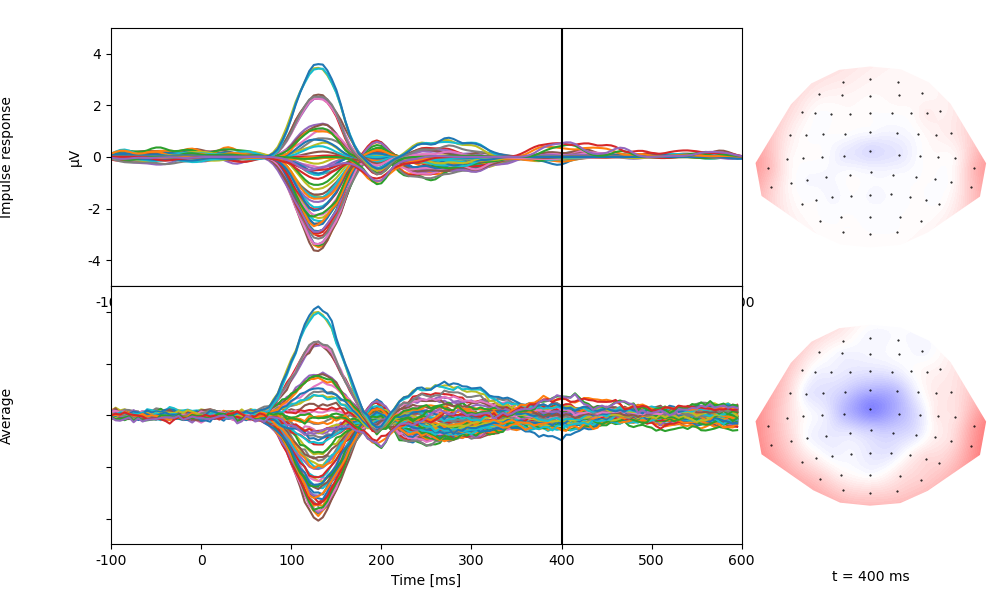## Categorial coding

Impulse predictors can be used like dummy codes in a regression model. Use one impulse to code for occurrence of any word (`any_word`), and a second impulse to code for unpredictable words only (`cloze`):

```any_word = epoch_impulse_predictor('eeg', 1, data=ds, name='any_word')
# effect code for cloze (1 for low cloze, -1 for high cloze)
cloze_code = Var.from_dict(ds['predictability'], {'high': 0, 'low': 1})
low_cloze = epoch_impulse_predictor('eeg', cloze_code, data=ds, name='low_cloze')

# plot the predictors for each trial
p = plot.UTS([any_word, low_cloze], '.case', stem=True)
```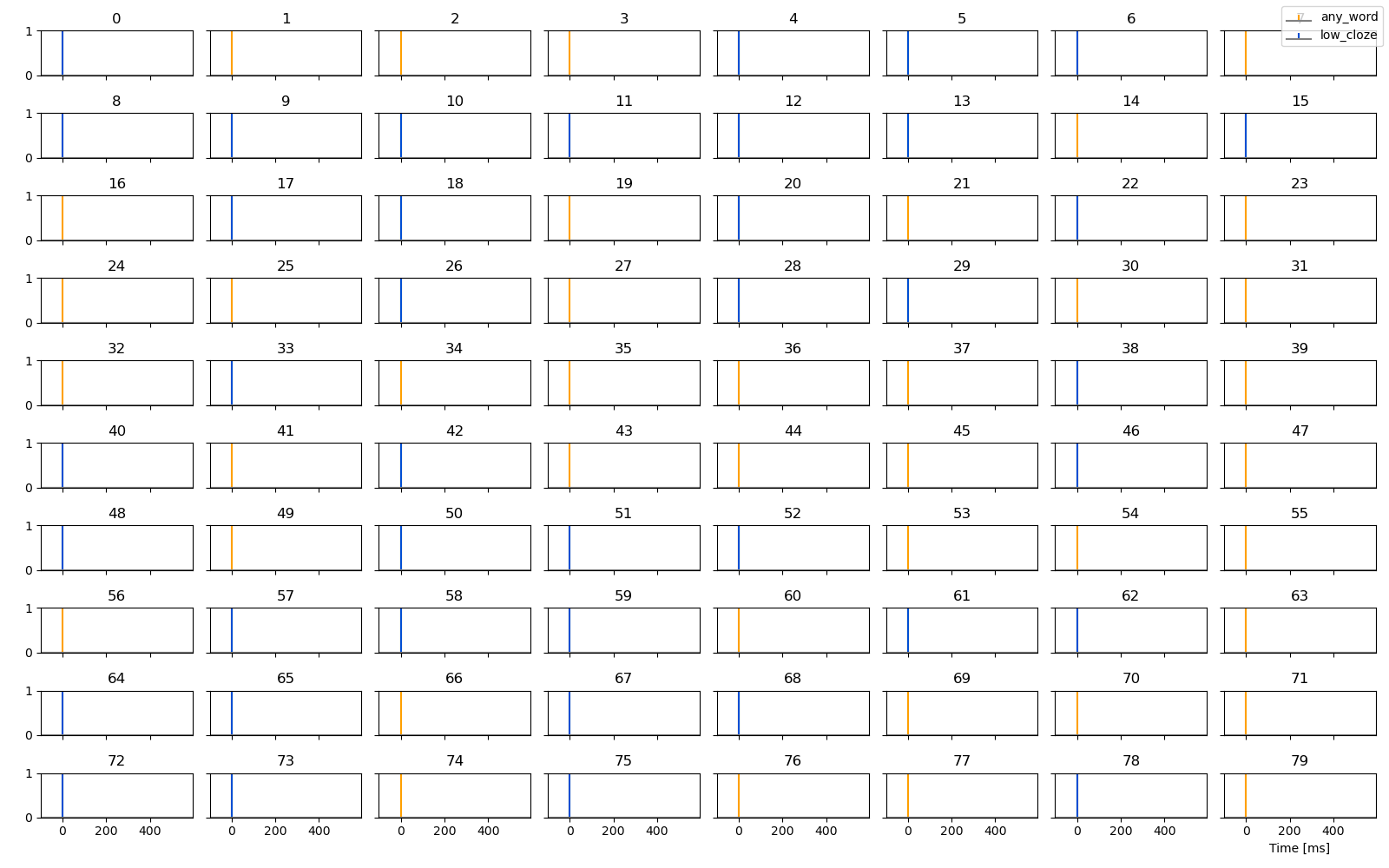Estimate response functions for these two predictors. Based on the coding, `any_word` reflects the response to predictable words, and `low_cloze` reflects how unpredictable words differ from predictable words:

```fit = boosting('eeg', [any_word, low_cloze], 0, 0.5, basis=0.050, model='predictability', data=ds, partitions=2, delta=0.01)
p = plot.TopoButterfly(fit.h, xlim=(-0.100, 0.600), t=0.400)
```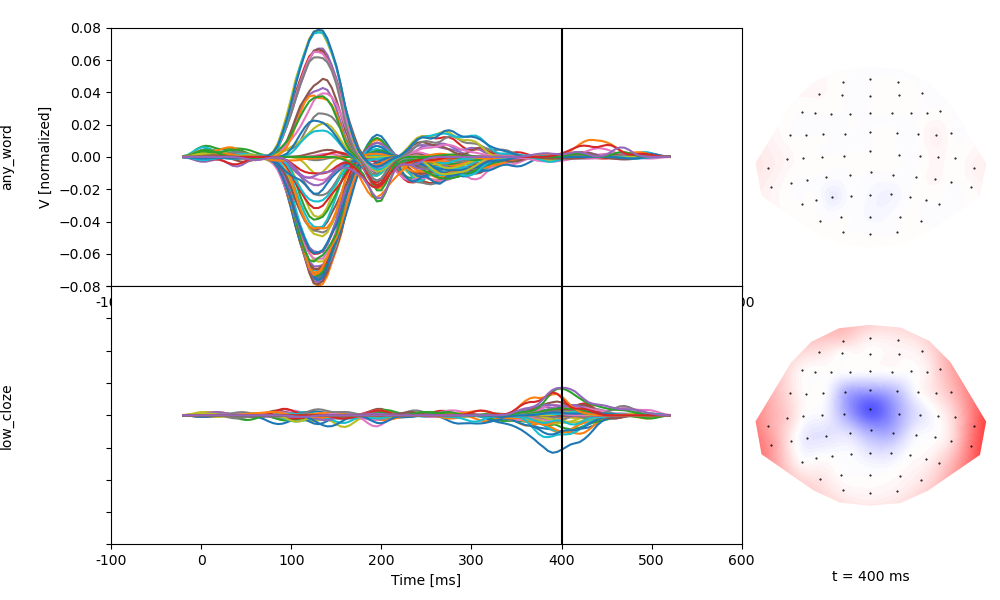## Continuous coding

Impulse predictors can similarly accommodate continuous variables:

```# effect code for cloze (1 for low cloze, -1 for high cloze)
n_chars = epoch_impulse_predictor('eeg', 'n_chars', data=ds, name='n_chars')
surprisal = epoch_impulse_predictor('eeg', '-numpy.log2(cloze)', data=ds, name='surprisal')

# plot the predictors for each trial
p = plot.UTS([n_chars, surprisal, any_word], '.case', stem=True)
```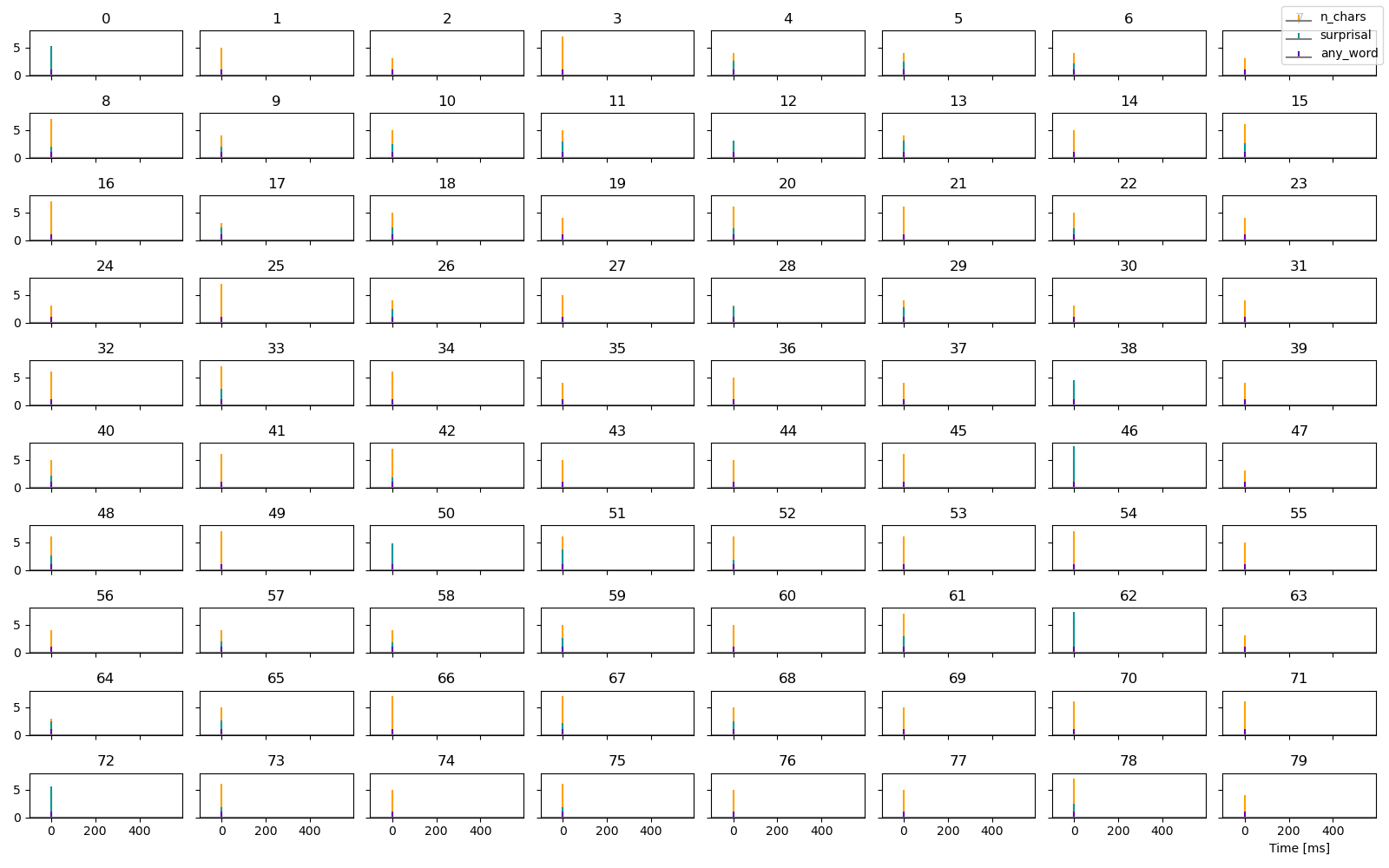Estimate response functions. Based on the coding, `any_word` reflects the hypothetical response to a words with 0 characters and 0 surprisal, whereas the two other predictors reflect the change in response by `n_chars` and surprisal:

```fit = boosting('eeg', [any_word, n_chars, surprisal], 0, 0.5, basis=0.050, model='predictability', data=ds, partitions=2, delta=0.01)
p = plot.TopoButterfly(fit.h, xlim=(-0.100, 0.600), t=0.400)
```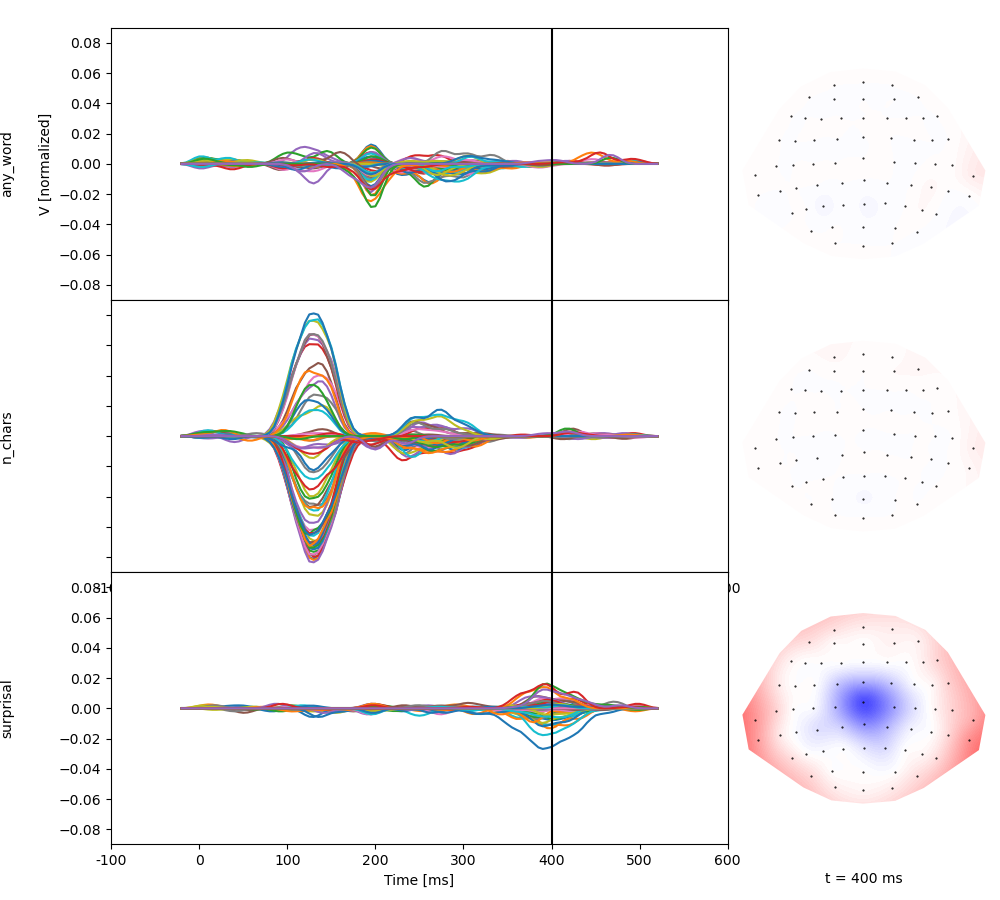Total running time of the script: (1 minutes 5.191 seconds)

Gallery generated by Sphinx-Gallery